GeeksforGeeks App
Open AppBrowser
Continue

# Dijkstra’s Algorithm for Adjacency List Representation | Greedy Algo-8

We recommend reading the following two posts as a prerequisite for this post.

We have discussed Dijkstra’s algorithm and its implementation for adjacency matrix representation of graphs. The time complexity for the matrix representation is O(V^2). In this post, O(ELogV) algorithm for adjacency list representation is discussed.
As discussed in the previous post, in Dijkstra’s algorithm, two sets are maintained, one set contains a list of vertices already included in SPT (Shortest Path Tree), and another set contains vertices not yet included. With adjacency list representation, all vertices of a graph can be traversed in O(V+E) time using BFS. The idea is to traverse all vertices of the graph using BFS and use a Min Heap to store the vertices not yet included in SPT (or the vertices for which the shortest distance is not finalized yet).  Min Heap is used as a priority queue to get the minimum distance vertex from a set of not yet included vertices. The time complexity of operations like extract-min and decrease-key value is O(LogV) for Min Heap.

Following are the detailed steps.

1. Create a Min Heap of size V where V is the number of vertices in the given graph. Every node of the min-heap contains the vertex number and distance value of the vertex.
2. Initialize Min Heap with source vertex as root (the distance value assigned to source vertex is 0). The distance value assigned to all other vertices is INF (infinite).
3. While Min Heap is not empty, do the following :
1. Extract the vertex with minimum distance value node from Min Heap. Let the extracted vertex be u.
2. For every adjacent vertex v of u, check if v is in Min Heap. If v is in Min Heap and the distance value is more than the weight of u-v plus the distance value of u, then update the distance value of v.

Let us understand with the following example. Let the given source vertex be 0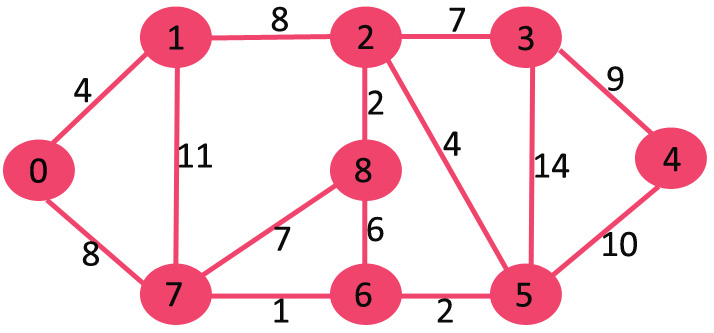Initially, the distance value of the source vertex is 0 and INF (infinite) for all other vertices. So source vertex is extracted from Min Heap and distance values of vertices adjacent to 0 (1 and 7) are updated. Min Heap contains all vertices except vertex 0.
The vertices in green color are the vertices for which minimum distances are finalized and are not in Min Heap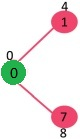Since the distance value of vertex 1 is minimum among all nodes in Min Heap, it is extracted from Min Heap and distance values of vertices adjacent to 1 are updated (distance is updated if the vertex is in Min Heap and distance through 1 is shorter than the previous distance). Min Heap contains all vertices except vertex 0 and 1.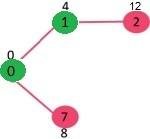Pick the vertex with a minimum distance value from the min-heap. Vertex 7 is picked. So min-heap now contains all vertices except 0, 1, and 7. Update the distance values of adjacent vertices of 7. The distance value of vertex 6 and 8 becomes finite (15 and 9 respectively).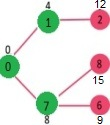Pick the vertex with a minimum distance from the min-heap. Vertex 6 is picked. So min-heap now contains all vertices except 0, 1, 7, and 6. Update the distance values of adjacent vertices of 6. The distance value of vertex 5 and 8 are updated.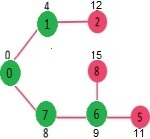The above steps are repeated till the min-heap doesn’t become empty. Finally, we get the following shortest-path tree.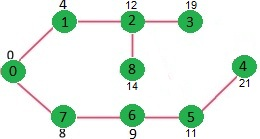Below is the implementation of the above approach:

## C++

 `// C / C++ program for Dijkstra's``// shortest path algorithm for adjacency``// list representation of graph``#include ``#include ``#include ` `// A structure to represent a``// node in adjacency list``struct` `AdjListNode``{``    ``int` `dest;``    ``int` `weight;``    ``struct` `AdjListNode* next;``};` `// A structure to represent``// an adjacency list``struct` `AdjList``{``    ` `   ``// Pointer to head node of list``   ``struct` `AdjListNode *head;``};` `// A structure to represent a graph.``// A graph is an array of adjacency lists.``// Size of array will be V (number of``// vertices in graph)``struct` `Graph``{``    ``int` `V;``    ``struct` `AdjList* array;``};` `// A utility function to create``// a new adjacency list node``struct` `AdjListNode* newAdjListNode(``                   ``int` `dest, ``int` `weight)``{``    ``struct` `AdjListNode* newNode =``            ``(``struct` `AdjListNode*)``      ``malloc``(``sizeof``(``struct` `AdjListNode));``    ``newNode->dest = dest;``    ``newNode->weight = weight;``    ``newNode->next = NULL;``    ``return` `newNode;``}` `// A utility function that creates``// a graph of V vertices``struct` `Graph* createGraph(``int` `V)``{``    ``struct` `Graph* graph = (``struct` `Graph*)``            ``malloc``(``sizeof``(``struct` `Graph));``    ``graph->V = V;` `    ``// Create an array of adjacency lists. ``    ``// Size of array will be V``    ``graph->array = (``struct` `AdjList*)``       ``malloc``(V * ``sizeof``(``struct` `AdjList));` `    ``// Initialize each adjacency list``    ``// as empty by making head as NULL``    ``for` `(``int` `i = 0; i < V; ++i)``        ``graph->array[i].head = NULL;` `    ``return` `graph;``}` `// Adds an edge to an undirected graph``void` `addEdge(``struct` `Graph* graph, ``int` `src,``                   ``int` `dest, ``int` `weight)``{``    ``// Add an edge from src to dest. ``    ``// A new node is added to the adjacency``    ``// list of src.  The node is``    ``// added at the beginning``    ``struct` `AdjListNode* newNode =``            ``newAdjListNode(dest, weight);``    ``newNode->next = graph->array[src].head;``    ``graph->array[src].head = newNode;` `    ``// Since graph is undirected,``    ``// add an edge from dest to src also``    ``newNode = newAdjListNode(src, weight);``    ``newNode->next = graph->array[dest].head;``    ``graph->array[dest].head = newNode;``}` `// Structure to represent a min heap node``struct` `MinHeapNode``{``    ``int`  `v;``    ``int` `dist;``};` `// Structure to represent a min heap``struct` `MinHeap``{``    ` `    ``// Number of heap nodes present currently``    ``int` `size;    ``  ` `    ``// Capacity of min heap``    ``int` `capacity; ``  ` `    ``// This is needed for decreaseKey()``    ``int` `*pos;   ``    ``struct` `MinHeapNode **array;``};` `// A utility function to create a``// new Min Heap Node``struct` `MinHeapNode* newMinHeapNode(``int` `v,``                                 ``int` `dist)``{``    ``struct` `MinHeapNode* minHeapNode =``           ``(``struct` `MinHeapNode*)``      ``malloc``(``sizeof``(``struct` `MinHeapNode));``    ``minHeapNode->v = v;``    ``minHeapNode->dist = dist;``    ``return` `minHeapNode;``}` `// A utility function to create a Min Heap``struct` `MinHeap* createMinHeap(``int` `capacity)``{``    ``struct` `MinHeap* minHeap =``         ``(``struct` `MinHeap*)``      ``malloc``(``sizeof``(``struct` `MinHeap));``    ``minHeap->pos = (``int` `*)``malloc``(``            ``capacity * ``sizeof``(``int``));``    ``minHeap->size = 0;``    ``minHeap->capacity = capacity;``    ``minHeap->array =``         ``(``struct` `MinHeapNode**)``                 ``malloc``(capacity *``       ``sizeof``(``struct` `MinHeapNode*));``    ``return` `minHeap;``}` `// A utility function to swap two``// nodes of min heap.``// Needed for min heapify``void` `swapMinHeapNode(``struct` `MinHeapNode** a,``                     ``struct` `MinHeapNode** b)``{``    ``struct` `MinHeapNode* t = *a;``    ``*a = *b;``    ``*b = t;``}` `// A standard function to``// heapify at given idx``// This function also updates``// position of nodes when they are swapped.``// Position is needed for decreaseKey()``void` `minHeapify(``struct` `MinHeap* minHeap,``                                  ``int` `idx)``{``    ``int` `smallest, left, right;``    ``smallest = idx;``    ``left = 2 * idx + 1;``    ``right = 2 * idx + 2;` `    ``if` `(left < minHeap->size &&``        ``minHeap->array[left]->dist <``         ``minHeap->array[smallest]->dist )``      ``smallest = left;` `    ``if` `(right < minHeap->size &&``        ``minHeap->array[right]->dist <``         ``minHeap->array[smallest]->dist )``      ``smallest = right;` `    ``if` `(smallest != idx)``    ``{``        ``// The nodes to be swapped in min heap``        ``MinHeapNode *smallestNode =``             ``minHeap->array[smallest];``        ``MinHeapNode *idxNode =``                 ``minHeap->array[idx];` `        ``// Swap positions``        ``minHeap->pos[smallestNode->v] = idx;``        ``minHeap->pos[idxNode->v] = smallest;` `        ``// Swap nodes``        ``swapMinHeapNode(&minHeap->array[smallest],``                         ``&minHeap->array[idx]);` `        ``minHeapify(minHeap, smallest);``    ``}``}` `// A utility function to check if``// the given minHeap is empty or not``int` `isEmpty(``struct` `MinHeap* minHeap)``{``    ``return` `minHeap->size == 0;``}` `// Standard function to extract``// minimum node from heap``struct` `MinHeapNode* extractMin(``struct` `MinHeap*``                                   ``minHeap)``{``    ``if` `(isEmpty(minHeap))``        ``return` `NULL;` `    ``// Store the root node``    ``struct` `MinHeapNode* root =``                   ``minHeap->array;` `    ``// Replace root node with last node``    ``struct` `MinHeapNode* lastNode =``         ``minHeap->array[minHeap->size - 1];``    ``minHeap->array = lastNode;` `    ``// Update position of last node``    ``minHeap->pos[root->v] = minHeap->size-1;``    ``minHeap->pos[lastNode->v] = 0;` `    ``// Reduce heap size and heapify root``    ``--minHeap->size;``    ``minHeapify(minHeap, 0);` `    ``return` `root;``}` `// Function to decreasekey dist value``// of a given vertex v. This function``// uses pos[] of min heap to get the``// current index of node in min heap``void` `decreaseKey(``struct` `MinHeap* minHeap,``                         ``int` `v, ``int` `dist)``{``    ``// Get the index of v in  heap array``    ``int` `i = minHeap->pos[v];` `    ``// Get the node and update its dist value``    ``minHeap->array[i]->dist = dist;` `    ``// Travel up while the complete``    ``// tree is not heapified.``    ``// This is a O(Logn) loop``    ``while` `(i && minHeap->array[i]->dist <``           ``minHeap->array[(i - 1) / 2]->dist)``    ``{``        ``// Swap this node with its parent``        ``minHeap->pos[minHeap->array[i]->v] =``                                      ``(i-1)/2;``        ``minHeap->pos[minHeap->array[``                             ``(i-1)/2]->v] = i;``        ``swapMinHeapNode(&minHeap->array[i], ``                 ``&minHeap->array[(i - 1) / 2]);` `        ``// move to parent index``        ``i = (i - 1) / 2;``    ``}``}` `// A utility function to check if a given vertex``// 'v' is in min heap or not``bool` `isInMinHeap(``struct` `MinHeap *minHeap, ``int` `v)``{``   ``if` `(minHeap->pos[v] < minHeap->size)``     ``return` `true``;``   ``return` `false``;``}` `// A utility function used to print the solution``void` `printArr(``int` `dist[], ``int` `n)``{``    ``printf``(``"Vertex   Distance from Source\n"``);``    ``for` `(``int` `i = 0; i < n; ++i)``        ``printf``(``"%d \t\t %d\n"``, i, dist[i]);``}` `// The main function that calculates``// distances of shortest paths from src to all``// vertices. It is a O(ELogV) function``void` `dijkstra(``struct` `Graph* graph, ``int` `src)``{``    ` `    ``// Get the number of vertices in graph``    ``int` `V = graph->V;``  ` `    ``// dist values used to pick``    ``// minimum weight edge in cut``    ``int` `dist[V];    ` `    ``// minHeap represents set E``    ``struct` `MinHeap* minHeap = createMinHeap(V);` `    ``// Initialize min heap with all``    ``// vertices. dist value of all vertices``    ``for` `(``int` `v = 0; v < V; ++v)``    ``{``        ``dist[v] = INT_MAX;``        ``minHeap->array[v] = newMinHeapNode(v,``                                      ``dist[v]);``        ``minHeap->pos[v] = v;``    ``}` `    ``// Make dist value of src vertex``    ``// as 0 so that it is extracted first``    ``minHeap->array[src] =``          ``newMinHeapNode(src, dist[src]);``    ``minHeap->pos[src]   = src;``    ``dist[src] = 0;``    ``decreaseKey(minHeap, src, dist[src]);` `    ``// Initially size of min heap is equal to V``    ``minHeap->size = V;` `    ``// In the following loop,``    ``// min heap contains all nodes``    ``// whose shortest distance``    ``// is not yet finalized.``    ``while` `(!isEmpty(minHeap))``    ``{``        ``// Extract the vertex with``        ``// minimum distance value``        ``struct` `MinHeapNode* minHeapNode =``                     ``extractMin(minHeap);``      ` `        ``// Store the extracted vertex number``        ``int` `u = minHeapNode->v;` `        ``// Traverse through all adjacent``        ``// vertices of u (the extracted``        ``// vertex) and update``        ``// their distance values``        ``struct` `AdjListNode* pCrawl =``                     ``graph->array[u].head;``        ``while` `(pCrawl != NULL)``        ``{``            ``int` `v = pCrawl->dest;` `            ``// If shortest distance to v is``            ``// not finalized yet, and distance to v``            ``// through u is less than its``            ``// previously calculated distance``            ``if` `(isInMinHeap(minHeap, v) &&``                      ``dist[u] != INT_MAX &&``              ``pCrawl->weight + dist[u] < dist[v])``            ``{``                ``dist[v] = dist[u] + pCrawl->weight;` `                ``// update distance``                ``// value in min heap also``                ``decreaseKey(minHeap, v, dist[v]);``            ``}``            ``pCrawl = pCrawl->next;``        ``}``    ``}` `    ``// print the calculated shortest distances``    ``printArr(dist, V);``}`  `// Driver program to test above functions``int` `main()``{``    ``// create the graph given in above figure``    ``int` `V = 9;``    ``struct` `Graph* graph = createGraph(V);``    ``addEdge(graph, 0, 1, 4);``    ``addEdge(graph, 0, 7, 8);``    ``addEdge(graph, 1, 2, 8);``    ``addEdge(graph, 1, 7, 11);``    ``addEdge(graph, 2, 3, 7);``    ``addEdge(graph, 2, 8, 2);``    ``addEdge(graph, 2, 5, 4);``    ``addEdge(graph, 3, 4, 9);``    ``addEdge(graph, 3, 5, 14);``    ``addEdge(graph, 4, 5, 10);``    ``addEdge(graph, 5, 6, 2);``    ``addEdge(graph, 6, 7, 1);``    ``addEdge(graph, 6, 8, 6);``    ``addEdge(graph, 7, 8, 7);` `    ``dijkstra(graph, 0);` `    ``return` `0;``}`

## Java

 `import` `java.io.*;``import` `java.util.*;` `class` `GFG {``    ``static` `class` `AdjListNode {``        ``int` `vertex, weight;` `        ``AdjListNode(``int` `v, ``int` `w)``        ``{``            ``vertex = v;``            ``weight = w;``        ``}``        ``int` `getVertex() { ``return` `vertex; }``        ``int` `getWeight() { ``return` `weight; }``    ``}` `    ``// Function to find the shortest distance of all the``    ``// vertices from the source vertex S.``    ``public` `static` `int``[] dijkstra(``        ``int` `V, ArrayList > graph,``        ``int` `src)``    ``{``        ``int``[] distance = ``new` `int``[V];``        ``for` `(``int` `i = ``0``; i < V; i++)``            ``distance[i] = Integer.MAX_VALUE;``        ``distance[src] = ``0``;` `        ``PriorityQueue pq = ``new` `PriorityQueue<>(``            ``(v1, v2) -> v1.getWeight() - v2.getWeight());``        ``pq.add(``new` `AdjListNode(src, ``0``));` `        ``while` `(pq.size() > ``0``) {``            ``AdjListNode current = pq.poll();` `            ``for` `(AdjListNode n :``                 ``graph.get(current.getVertex())) {``                ``if` `(distance[current.getVertex()]``                        ``+ n.getWeight()``                    ``< distance[n.getVertex()]) {``                    ``distance[n.getVertex()]``                        ``= n.getWeight()``                          ``+ distance[current.getVertex()];``                    ``pq.add(``new` `AdjListNode(``                        ``n.getVertex(),``                        ``distance[n.getVertex()]));``                ``}``            ``}``        ``}``        ``// If you want to calculate distance from source to``        ``// a particular target, you can return``        ``// distance[target]``        ``return` `distance;``    ``}` `    ``public` `static` `void` `main(String[] args)``    ``{``        ``int` `V = ``9``;``        ``ArrayList > graph``            ``= ``new` `ArrayList<>();``        ``for` `(``int` `i = ``0``; i < V; i++) {``            ``graph.add(``new` `ArrayList<>());``        ``}``        ``int` `source = ``0``;``        ``graph.get(``0``).add(``new` `AdjListNode(``1``, ``4``));``        ``graph.get(``0``).add(``new` `AdjListNode(``7``, ``8``));``        ``graph.get(``1``).add(``new` `AdjListNode(``2``, ``8``));``        ``graph.get(``1``).add(``new` `AdjListNode(``7``, ``11``));``        ``graph.get(``1``).add(``new` `AdjListNode(``0``, ``7``));``        ``graph.get(``2``).add(``new` `AdjListNode(``1``, ``8``));``        ``graph.get(``2``).add(``new` `AdjListNode(``3``, ``7``));``        ``graph.get(``2``).add(``new` `AdjListNode(``8``, ``2``));``        ``graph.get(``2``).add(``new` `AdjListNode(``5``, ``4``));``        ``graph.get(``3``).add(``new` `AdjListNode(``2``, ``7``));``        ``graph.get(``3``).add(``new` `AdjListNode(``4``, ``9``));``        ``graph.get(``3``).add(``new` `AdjListNode(``5``, ``14``));``        ``graph.get(``4``).add(``new` `AdjListNode(``3``, ``9``));``        ``graph.get(``4``).add(``new` `AdjListNode(``5``, ``10``));``        ``graph.get(``5``).add(``new` `AdjListNode(``4``, ``10``));``        ``graph.get(``5``).add(``new` `AdjListNode(``6``, ``2``));``        ``graph.get(``6``).add(``new` `AdjListNode(``5``, ``2``));``        ``graph.get(``6``).add(``new` `AdjListNode(``7``, ``1``));``        ``graph.get(``6``).add(``new` `AdjListNode(``8``, ``6``));``        ``graph.get(``7``).add(``new` `AdjListNode(``0``, ``8``));``        ``graph.get(``7``).add(``new` `AdjListNode(``1``, ``11``));``        ``graph.get(``7``).add(``new` `AdjListNode(``6``, ``1``));``        ``graph.get(``7``).add(``new` `AdjListNode(``8``, ``7``));``        ``graph.get(``8``).add(``new` `AdjListNode(``2``, ``2``));``        ``graph.get(``8``).add(``new` `AdjListNode(``6``, ``6``));``        ``graph.get(``8``).add(``new` `AdjListNode(``7``, ``1``));` `        ``int``[] distance = dijkstra(V, graph, source);``        ``// Printing the Output``        ``System.out.println(``"Vertex  "``                           ``+ ``"  Distance from Source"``);``        ``for` `(``int` `i = ``0``; i < V; i++) {``            ``System.out.println(i + ``"             "``                               ``+ distance[i]);``        ``}``    ``}``}`

## Python3

 `# A Python program for Dijkstra's shortest``# path algorithm for adjacency``# list representation of graph` `from` `collections ``import` `defaultdict``import` `sys`  `class` `Heap():` `    ``def` `__init__(``self``):``        ``self``.array ``=` `[]``        ``self``.size ``=` `0``        ``self``.pos ``=` `[]` `    ``def` `newMinHeapNode(``self``, v, dist):``        ``minHeapNode ``=` `[v, dist]``        ``return` `minHeapNode` `    ``# A utility function to swap two nodes``    ``# of min heap. Needed for min heapify``    ``def` `swapMinHeapNode(``self``, a, b):``        ``t ``=` `self``.array[a]``        ``self``.array[a] ``=` `self``.array[b]``        ``self``.array[b] ``=` `t` `    ``# A standard function to heapify at given idx``    ``# This function also updates position of nodes``    ``# when they are swapped.Position is needed``    ``# for decreaseKey()``    ``def` `minHeapify(``self``, idx):``        ``smallest ``=` `idx``        ``left ``=` `2``*``idx ``+` `1``        ``right ``=` `2``*``idx ``+` `2` `        ``if` `(left < ``self``.size ``and``           ``self``.array[left][``1``]``            ``< ``self``.array[smallest][``1``]):``            ``smallest ``=` `left` `        ``if` `(right < ``self``.size ``and``           ``self``.array[right][``1``]``            ``< ``self``.array[smallest][``1``]):``            ``smallest ``=` `right` `        ``# The nodes to be swapped in min``        ``# heap if idx is not smallest``        ``if` `smallest !``=` `idx:` `            ``# Swap positions``            ``self``.pos[``self``.array[smallest][``0``]] ``=` `idx``            ``self``.pos[``self``.array[idx][``0``]] ``=` `smallest` `            ``# Swap nodes``            ``self``.swapMinHeapNode(smallest, idx)` `            ``self``.minHeapify(smallest)` `    ``# Standard function to extract minimum``    ``# node from heap``    ``def` `extractMin(``self``):` `        ``# Return NULL wif heap is empty``        ``if` `self``.isEmpty() ``=``=` `True``:``            ``return` `        ``# Store the root node``        ``root ``=` `self``.array[``0``]` `        ``# Replace root node with last node``        ``lastNode ``=` `self``.array[``self``.size ``-` `1``]``        ``self``.array[``0``] ``=` `lastNode` `        ``# Update position of last node``        ``self``.pos[lastNode[``0``]] ``=` `0``        ``self``.pos[root[``0``]] ``=` `self``.size ``-` `1` `        ``# Reduce heap size and heapify root``        ``self``.size ``-``=` `1``        ``self``.minHeapify(``0``)` `        ``return` `root` `    ``def` `isEmpty(``self``):``        ``return` `True` `if` `self``.size ``=``=` `0` `else` `False` `    ``def` `decreaseKey(``self``, v, dist):` `        ``# Get the index of v in  heap array` `        ``i ``=` `self``.pos[v]` `        ``# Get the node and update its dist value``        ``self``.array[i][``1``] ``=` `dist` `        ``# Travel up while the complete tree is``        ``# not heapified. This is a O(Logn) loop``        ``while` `(i > ``0` `and` `self``.array[i][``1``] <``                  ``self``.array[(i ``-` `1``) ``/``/` `2``][``1``]):` `            ``# Swap this node with its parent``            ``self``.pos[ ``self``.array[i][``0``] ] ``=` `(i``-``1``)``/``/``2``            ``self``.pos[ ``self``.array[(i``-``1``)``/``/``2``][``0``] ] ``=` `i``            ``self``.swapMinHeapNode(i, (i ``-` `1``)``/``/``2` `)` `            ``# move to parent index``            ``i ``=` `(i ``-` `1``) ``/``/` `2``;` `    ``# A utility function to check if a given``    ``# vertex 'v' is in min heap or not``    ``def` `isInMinHeap(``self``, v):` `        ``if` `self``.pos[v] < ``self``.size:``            ``return` `True``        ``return` `False`  `def` `printArr(dist, n):``    ``print` `(``"Vertex\tDistance from source"``)``    ``for` `i ``in` `range``(n):``        ``print` `(``"%d\t\t%d"` `%` `(i,dist[i]))`  `class` `Graph():` `    ``def` `__init__(``self``, V):``        ``self``.V ``=` `V``        ``self``.graph ``=` `defaultdict(``list``)` `    ``# Adds an edge to an undirected graph``    ``def` `addEdge(``self``, src, dest, weight):` `        ``# Add an edge from src to dest.  A new node``        ``# is added to the adjacency list of src. The``        ``# node is added at the beginning. The first``        ``# element of the node has the destination``        ``# and the second elements has the weight``        ``newNode ``=` `[dest, weight]``        ``self``.graph[src].insert(``0``, newNode)` `        ``# Since graph is undirected, add an edge``        ``# from dest to src also``        ``newNode ``=` `[src, weight]``        ``self``.graph[dest].insert(``0``, newNode)` `    ``# The main function that calculates distances``    ``# of shortest paths from src to all vertices.``    ``# It is a O(ELogV) function``    ``def` `dijkstra(``self``, src):` `        ``V ``=` `self``.V  ``# Get the number of vertices in graph``        ``dist ``=` `[]   ``# dist values used to pick minimum``                    ``# weight edge in cut` `        ``# minHeap represents set E``        ``minHeap ``=` `Heap()` `        ``#  Initialize min heap with all vertices.``        ``# dist value of all vertices``        ``for` `v ``in` `range``(V):``            ``dist.append(``1e7``)``            ``minHeap.array.append( minHeap.``                                ``newMinHeapNode(v, dist[v]))``            ``minHeap.pos.append(v)` `        ``# Make dist value of src vertex as 0 so``        ``# that it is extracted first``        ``minHeap.pos[src] ``=` `src``        ``dist[src] ``=` `0``        ``minHeap.decreaseKey(src, dist[src])` `        ``# Initially size of min heap is equal to V``        ``minHeap.size ``=` `V;` `        ``# In the following loop,``        ``# min heap contains all nodes``        ``# whose shortest distance is not yet finalized.``        ``while` `minHeap.isEmpty() ``=``=` `False``:` `            ``# Extract the vertex``            ``# with minimum distance value``            ``newHeapNode ``=` `minHeap.extractMin()``            ``u ``=` `newHeapNode[``0``]` `            ``# Traverse through all adjacent vertices of``            ``# u (the extracted vertex) and update their``            ``# distance values``            ``for` `pCrawl ``in` `self``.graph[u]:` `                ``v ``=` `pCrawl[``0``]` `                ``# If shortest distance to v is not finalized``                ``# yet, and distance to v through u is less``                ``# than its previously calculated distance``                ``if` `(minHeap.isInMinHeap(v) ``and``                     ``dist[u] !``=` `1e7` `and` `\``                   ``pCrawl[``1``] ``+` `dist[u] < dist[v]):``                        ``dist[v] ``=` `pCrawl[``1``] ``+` `dist[u]` `                        ``# update distance value``                        ``# in min heap also``                        ``minHeap.decreaseKey(v, dist[v])` `        ``printArr(dist,V)`  `# Driver program to test the above functions``graph ``=` `Graph(``9``)``graph.addEdge(``0``, ``1``, ``4``)``graph.addEdge(``0``, ``7``, ``8``)``graph.addEdge(``1``, ``2``, ``8``)``graph.addEdge(``1``, ``7``, ``11``)``graph.addEdge(``2``, ``3``, ``7``)``graph.addEdge(``2``, ``8``, ``2``)``graph.addEdge(``2``, ``5``, ``4``)``graph.addEdge(``3``, ``4``, ``9``)``graph.addEdge(``3``, ``5``, ``14``)``graph.addEdge(``4``, ``5``, ``10``)``graph.addEdge(``5``, ``6``, ``2``)``graph.addEdge(``6``, ``7``, ``1``)``graph.addEdge(``6``, ``8``, ``6``)``graph.addEdge(``7``, ``8``, ``7``)``graph.dijkstra(``0``)` `# This code is contributed by Divyanshu Mehta`

## C#

 `// C# program for Dijkstra's``// shortest path algorithm for adjacency``// list representation of graph``using` `System;``using` `System.Collections.Generic;``using` `System.Linq;` `public` `class` `AdjListNode : IComparable {``  ``private` `int` `vertex, weight;``  ``public` `AdjListNode(``int` `v, ``int` `w)``  ``{``    ``vertex = v;``    ``weight = w;``  ``}``  ``public` `int` `getVertex() { ``return` `vertex; }``  ``public` `int` `getWeight() { ``return` `weight; }``  ``public` `int` `CompareTo(AdjListNode other)``  ``{``    ``return` `weight - other.weight;``  ``}``}` `class` `GFG {``  ``// Function to find the shortest distance of all the``  ``// vertices from the source vertex S.``  ``public` `static` `int``[] dijkstra(``    ``int` `V, List > graph, ``int` `src)``  ``{``    ``int``[] distance = ``new` `int``[V];``    ``for` `(``int` `i = 0; i < V; i++)``      ``distance[i] = Int32.MaxValue;``    ``distance[src] = 0;` `    ``SortedSet pq``      ``= ``new` `SortedSet();``    ``pq.Add(``new` `AdjListNode(src, 0));` `    ``while` `(pq.Count > 0) {``      ``AdjListNode current = pq.First();``      ``pq.Remove(current);` `      ``foreach``(``        ``AdjListNode n ``in` `graph[current.getVertex()])``      ``{``        ``if` `(distance[current.getVertex()]``            ``+ n.getWeight()``            ``< distance[n.getVertex()]) {``          ``distance[n.getVertex()]``            ``= n.getWeight()``            ``+ distance[current.getVertex()];``          ``pq.Add(``new` `AdjListNode(``            ``n.getVertex(),``            ``distance[n.getVertex()]));``        ``}``      ``}``    ``}``    ``// If you want to calculate distance from source to``    ``// a particular target, you can return``    ``// distance[target]``    ``return` `distance;``  ``}` `  ``static` `void` `Main(``string``[] args)``  ``{``    ``int` `V = 9;``    ``List > graph``      ``= ``new` `List >();``    ``for` `(``int` `i = 0; i < V; i++) {``      ``graph.Add(``new` `List());``    ``}``    ``int` `source = 0;``    ``graph.Add(``new` `AdjListNode(1, 4));``    ``graph.Add(``new` `AdjListNode(7, 8));``    ``graph.Add(``new` `AdjListNode(2, 8));``    ``graph.Add(``new` `AdjListNode(7, 11));``    ``graph.Add(``new` `AdjListNode(0, 7));``    ``graph.Add(``new` `AdjListNode(1, 8));``    ``graph.Add(``new` `AdjListNode(3, 7));``    ``graph.Add(``new` `AdjListNode(8, 2));``    ``graph.Add(``new` `AdjListNode(5, 4));``    ``graph.Add(``new` `AdjListNode(2, 7));``    ``graph.Add(``new` `AdjListNode(4, 9));``    ``graph.Add(``new` `AdjListNode(5, 14));``    ``graph.Add(``new` `AdjListNode(3, 9));``    ``graph.Add(``new` `AdjListNode(5, 10));``    ``graph.Add(``new` `AdjListNode(4, 10));``    ``graph.Add(``new` `AdjListNode(6, 2));``    ``graph.Add(``new` `AdjListNode(5, 2));``    ``graph.Add(``new` `AdjListNode(7, 1));``    ``graph.Add(``new` `AdjListNode(8, 6));``    ``graph.Add(``new` `AdjListNode(0, 8));``    ``graph.Add(``new` `AdjListNode(1, 11));``    ``graph.Add(``new` `AdjListNode(6, 1));``    ``graph.Add(``new` `AdjListNode(8, 7));``    ``graph.Add(``new` `AdjListNode(2, 2));``    ``graph.Add(``new` `AdjListNode(6, 6));``    ``graph.Add(``new` `AdjListNode(7, 1));` `    ``int``[] distance = dijkstra(V, graph, source);``    ``// Printing the Output``    ``Console.WriteLine(``"Vertex "``                      ``+ ``" Distance from Source"``);``    ``for` `(``int` `i = 0; i < V; i++) {``      ``Console.WriteLine(``        ``"{0}             {1}"``, i,``        ``distance[i]);``    ``}``  ``}``}` `// This code is contributed by cavi4762.`

## Javascript

 `// javascript program for Dijkstra's``// shortest path algorithm for adjacency``// list representation of graph`  `// A structure to represent a``// node in adjacency list``class AdjListNode {``  ``constructor(dest, weight) {``    ``this``.dest = dest;``    ``this``.weight = weight;``  ``}``}``// Function to find the shortest distance of all the``// vertices from the source vertex S.``function` `dijkstra(V, graph, source) {``  ``let distance = [];``  ``let visited = [];` `  ``for` `(let i = 0; i < V; i++) {``    ``distance.push(Infinity);``    ``visited.push(``false``);``  ``}` `  ``distance = 0;` `  ``for` `(let i = 0; i < V - 1; i++) {``    ``let u = getMinDistanceVertex(distance, visited);``    ``visited[u] = ``true``;` `    ``for` `(let j = 0; j < graph[u].length; j++) {``      ``let v = graph[u][j].dest;``      ``let weight = graph[u][j].weight;``      ``if` `(!visited[v] && distance[u] !== Infinity && distance[u] + weight < distance[v]) {``        ``distance[v] = distance[u] + weight;``      ``}``    ``}``  ``}` `  ``// If you want to calculate distance from source to``  ``// a particular target, you can return``  ``// distance[target]``  ``return` `distance;``}` `function` `getMinDistanceVertex(distance, visited) {``  ``let minDistance = Infinity;``  ``let minIndex = -1;` `  ``for` `(let i = 0; i < distance.length; i++) {``    ``if` `(!visited[i] && distance[i] <= minDistance) {``      ``minDistance = distance[i];``      ``minIndex = i;``    ``}``  ``}` `  ``return` `minIndex;``}``// create the graph given in above figure``const V = 9;``let graph = [];` `for` `(let i = 0; i < V; i++) {``  ``graph.push([]);``}` `let source = 0;` `graph.push(``new` `AdjListNode(1, 4));``graph.push(``new` `AdjListNode(7, 8));``graph.push(``new` `AdjListNode(2, 8));``graph.push(``new` `AdjListNode(7, 11));``graph.push(``new` `AdjListNode(0, 7));``graph.push(``new` `AdjListNode(1, 8));``graph.push(``new` `AdjListNode(3, 7));``graph.push(``new` `AdjListNode(8, 2));``graph.push(``new` `AdjListNode(5, 4));``graph.push(``new` `AdjListNode(2, 7));``graph.push(``new` `AdjListNode(4, 9));``graph.push(``new` `AdjListNode(5, 14));``graph.push(``new` `AdjListNode(3, 9));``graph.push(``new` `AdjListNode(5, 10));``graph.push(``new` `AdjListNode(4, 10));``graph.push(``new` `AdjListNode(6, 2));``graph.push(``new` `AdjListNode(5, 2));``graph.push(``new` `AdjListNode(7, 1));``graph.push(``new` `AdjListNode(8, 6));``graph.push(``new` `AdjListNode(0, 8));``graph.push(``new` `AdjListNode(1, 11));``graph.push(``new` `AdjListNode(6, 1));``graph.push(``new` `AdjListNode(8, 7));``graph.push(``new` `AdjListNode(2, 2));``graph.push(``new` `AdjListNode(6, 6));``graph.push(``new` `AdjListNode(7, 1));` `let distance = dijkstra(V, graph, source);``// Printing the Output``console.log(``"Vertex Distance from Source"``);``for` `(let i = 0; i < V; i++) {``    ``console.log(i + ``" \t\t "` `+ distance[i]);``}` `// This code is contributed by shivhack999`

Output

```Vertex   Distance from Source
0          0
1          4
2          12
3          19
4          21
5          11
6          9
7          8
8          14```

Time Complexity: The time complexity of the above code/algorithm looks O(V^2) as there are two nested while loops. If we take a closer look, we can observe that the statements in the inner loop are executed O(V+E) times (similar to BFS). The inner loop has decreaseKey() operation which takes O(LogV) time. So overall time complexity is O(E+V)*O(LogV) which is O((E+V)*LogV) = O(ELogV)
Note that the above code uses Binary Heap for Priority Queue implementation. Time complexity can be reduced to O(E + VLogV) using Fibonacci Heap. The reason is, that Fibonacci Heap takes O(1) time for decrease-key operation while Binary Heap takes O(Logn) time.

Space Complexity: O(V)

The space complexity of Dijkstra’s algorithm is O(V) as we maintain two priority queues or heaps (in case of binary heap). The heap stores the nodes which are not yet included in SPT (shortest path tree). We also maintain an array to store the distance values of each node.
Notes:

1. The code calculates the shortest distance but doesn’t calculate the path information. We can create a parent array, update the parent array when distance is updated (like prim’s implementation), and use it to show the shortest path from source to different vertices.
2. The code is for undirected graphs, same Dijkstra function can be used for directed graphs also.
3. The code finds the shortest distances from the source to all vertices. If we are interested only in the shortest distance from the source to a single target, we can break the for loop when the picked minimum distance vertex is equal to the target (Step 3.a of an algorithm).
4. Dijkstra’s algorithm doesn’t work for graphs with negative weight edges. For graphs with negative weight edges, Bellman–Ford algorithm can be used, we will soon be discussing it as a separate post.
Printing Paths in Dijkstra’s Shortest Path Algorithm
Dijkstra’s shortest path algorithm using set in STL